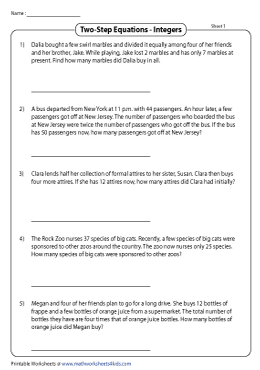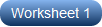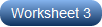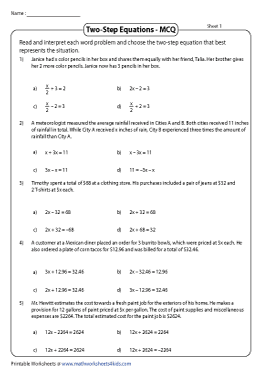# Systems of equations word problems worksheet pdf## Word Problems

Word Problems Worksheet 1 – This 6 problem algebra worksheet will help you practice creating and solving systems of equations to represent real-life situations. You will use the “elimination” method to eliminate variables from standard form equations.
Word Problems Worksheet 1 RTF
Word Problems Worksheet 1 PDF

Word Problems Worksheet 2 – This 6 problem algebra worksheet will help you practice creating and solving systems of equations to represent real-life situations. You will use the “elimination” method to eliminate variables from standard form equations.
Word Problems Worksheet 2 RTF
Word Problems Worksheet 2 PDF

Word Problems Worksheet 3 – This 6 problem algebra worksheet will help you practice creating and solving systems of equations to represent real-life situations. Most of the problems involve money, so make sure you’re ready for some decimals.
Word Problems Worksheet 3 RTF
Word Problems Worksheet 3 PDF

Word Problems Worksheet 4 – This 6 problem algebra worksheet will help you practice creating and solving systems of equations to represent real-life situations. Most of the problems involve money, and a few distractors are introduced.
Word Problems Worksheet 4 RTF
Word Problems Worksheet 4 PDF

Word Problems Worksheet 5 – This 8 problem algebra worksheet features more abstract word problems like “The sum of x and y is 42.  the difference of x and y is 13.  Find x and y.” There are a few negative integers, so be careful!
Word Problems Worksheet 5 RTF
Word Problems Worksheet 5 PDF

Word Problems Worksheet 6 – This 8 problem algebra worksheet features more abstract word problems like “The sum of  twice a number, x, and twice another number, y, is 118.  The value of y is one less than twice the value of x.  Find x and y.” One of the problems even has an infinite number of solutions!
Word Problems Worksheet 6 RTF
Word Problems Worksheet 6 PDF

These free systems of equationsworksheets will help you practice solving real-life systems of equations using the “elimination” method.  You will  need to create and solve a system of equations to represent each situation.  The exercises can also be solved using other algebraic methods if you choose.

This is a progressive series that starts simple with problems involving buying movie tickets and collecting for fundraisers.  Eventually the negative numbers, decimals, the distributive property and “the opposite of x” come into play.

Each worksheet will help students master Common Core skills in the Algebra strand.  They are great for ambitious students in pre-algebra or algebra classes.

These free elmination worksheets are printable and available in a variety of formats.  Each sheet includes an example to help you get started.  Of course, answer keys are provided as well.

Sours: http://imathworksheets.com/algebra-worksheets-page/systems-of-equations/elimination/word-problems/

## Word Problems

Word Problems Worksheet 1 – This 6 problem algebra worksheet will help you practice solving real-life systems of equations problems using the “substitution” method.  All of the coefficients and answers are positive integers.
Word Problems Worksheet 1 RTF
Word Problems Worksheet 1 PDF

Word Problems Worksheet 2 – This 6 problem algebra worksheet will help you practice solving real life systems of equations problems using the “substitution” method. Some of the substitution involves utilizing the distributive property.
Word Problems Worksheet 2 RTF
Word Problems Worksheet 2 PDF

Word Problems Worksheet 3 – This 6 problem algebra worksheet will help you practice solving real life systems of equations problems using the “substitution” method. Most of the problems involve money and require the use of the distributive property.
Word Problems Worksheet 3 RTF
Word Problems Worksheet 3 PDF

Word Problems Worksheet 4 – This 6 problem algebra worksheet will help you practice solving real life systems of equations problems using the “substitution” method. Most of the problems involve money. A few twists are incorporated, so read carefully!
Word Problems Worksheet 4 RTF
Word Problems Worksheet 4 PDF

Word Problems Worksheet 5 – This 8 problem algebra worksheet features more abstract word problems like “The sum of x and y is 42.  y is 12 less than x.  Find x and y.”  There are quite a few negative integers, so be careful!
Word Problems Worksheet 5 RTF
Word Problems Worksheet 5 PDF

Word Problems Worksheet 6 – This 8 problem algebra worksheet features more abstract word problems like “The sum of  three times a number, x, and half of another number, y, is 96.  y is 60 less than x.  Find x and y.” One of the problems even has an infinite number of solutions!
Word Problems Worksheet 6 RTF
Word Problems Worksheet 6 PDF

These free systems of equationsworksheets will help you practice solving real-life systems of equations using the “substitution” method.  You will  need to create and solve a system of equations to represent each situation.  The exercises can also be solved using other algebraic methods if you choose.

This is a progressive series that starts simple with problems involving buying pizza and figuring out ages.  Eventually the distributive property and “the opposite of x” come into play.  Watch out for those perimeter problems!

Each worksheet will help students master Common Core skills in the Algebra strand.  They are great for ambitious students in pre-algebra or algebra classes.

These free substitution worksheets are printable and available in a variety of formats.  Each sheet includes an example to help you get started.  Of course, answer keys are provided as well.

Sours: http://imathworksheets.com/algebra-worksheets-page/systems-of-equations/substitution/word-problems/

## Equation Word Problems Worksheets

This compilation of a meticulously drafted equation word problems worksheets is designed to get students to write and solve a variety of one-step, two-step and multi-step equations that involve integers, fractions, and decimals. These worksheets are best suited for students in grade 6 through high school. Click on the 'Free' icons to sample our handouts.Two-Step Equation Word Problems: Integers

Interpret this set of word problems that require two-step operations to solve the equations. Each printable worksheet has five word problems ideal for 6th grade, 7th grade, and 8th grade students.

••••MCQ - Two-Step Equation Word Problems

Pick the correct two-step equation that best matches word problems presented here. Evaluate the ability of students to solve two-step equations with this array of MCQ worksheets.

••••Multi-Step Equation Word Problems: Integers

Read each multi-step word problem in these high school pdf worksheets and set up the equation. Solve and find the value of the unknown. More than two steps are required to solve the problems.

••••Sours: https://www.mathworksheets4kids.com/equation-word-problems.php
Systems of Equations - Word problems \u0026 graphing

.

## Pdf systems of equations word problems worksheet

.

Systems of Equations Word Problems - 11 Examples

.

### You will also be interested:

.

816 817 818 819 820